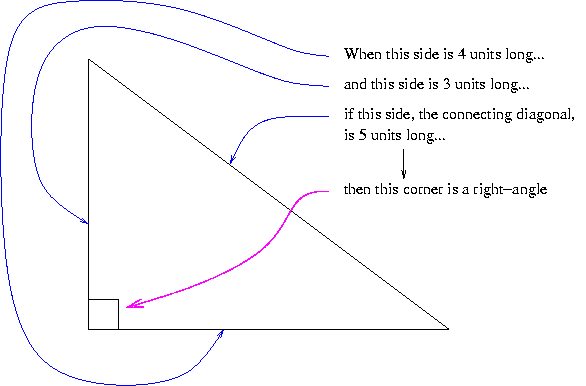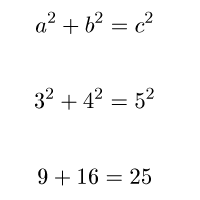# The 3:4:5 rule used in the construction trades

The "3 4 5 rule" is widely known and used in the construction trades.

It is used to obtain a right-angle ("squareness") between what is of interest.

Here is a pictorial representation of how the "3 4 5 rule" works:You often use it like this: you measure and mark off 4 units along one object and 3 units along the other object at roughly 90degrees to the first. Then you measure the diagonal directly connecting the two marks and adjust the angle between the objects until the diagonal connecting the marks is 5 units long. When this is made so, the angle between the two objects is a right-angle.

Commonly you are using a tape-measure when using the "3 4 5 rule". A typical use is when marking out chalk lines on a level concrete floor which will guide how you lay out the steels of a major construction.

## For the mathematically-minded

If you know Pythagoras, you can see this makes sense(look up "Pythagoras" yourself for full explanation!)

(R. Smith, 19 January 2008)